• MATLAB与VC-利用VC 调用matlab数学库和图形库的.rar 上传ＭＡＴＬＡＢ与ＶＣ的接口设计，及在利用ＶＣ调用ＭＡＴＬＡＢ的一些资料，希望对大家有用！
• 利用VC调用MATLAB实现欧拉公式运算，具有很好的实践，操作能力
• 本例子是Visual Studio2015调用Matlab2016生成的动态链接库的例子，实验运行通过，在运行前确保自己安装了Matlab运行时。
• 里面内含 matalb的m文件 可以直接运行，有两个例子，一个是简单的加法 ，另一个是输入输出矩阵。
• vc6通过代码 调用 matlab编译生成的com组件 mfile -> com组件 -> vc6 exe
• VC调用matlab技术，很好的程序，简单实用！
• 我的环境 matlab7.0， vs2012.执行mex -setup 和mbuild -setup 是选的vc6，vs执行命令的时候不可见 参考1：http://www.ilovematlab.cn/thread-205148-1-1.html ... 调用matlab动态库，
我的环境 matlab7.0， vs2012.执行mex -setup 和mbuild -setup 是选的vc6，vs执行命令的时候不可见
http://blog.csdn.net/nupt123456789/article/details/7463612
调用matlab动态库，使用matlab2010b可以，使用matlab7.0不可以，好像是7.0的问题，有大神碰到过可以指教一下哦。谢谢

//以上是调用动态库
//以下是调用matlab的figure画图
参考2：http://blog.csdn.net/u010177286/article/details/37561113 //一些添加注释的方法
参考3：http://blog.csdn.net/feichizhongwu888/article/details/47277565 //引擎的函数介绍，妻例子我没编译过
参考4：http://blog.sina.com.cn/s/blog_8e6bfecf010126pg.html //这个例子的数据是写好的无法传自己想要的数据，主要可以参考 他的工程的配置方法。
参考5：http://www.tuicool.com/articles/JZzmmiB //这个不错，有一点小问题，engClose(ep);要重写，可能是网页格式的问题，非常感谢
我的例子如下：
void CMFCmtalabDlg::OnBnClickedOk2()
{

Engine* ep;

mxArray *x1 = NULL;

mxArray *y1 = NULL;

mxArray *x2 = NULL;

mxArray *y2 = NULL;

ep = engOpen(NULL);

if( ep==NULL )

{

exit(-1);

}

//engOutputBuffer(ep, buffer, BUFFER_SIZE);

printf("Init Success");

double x = { 2.0, 4.5,6.7,8.4,9.1 };

double y = { 3.3,4.7,9.6,15.6,21.3 };

double xArr = { 1.0,2.0,3.0,4.0,5.0 };

double yArr = { 4.0,8.0,12.0,16.0,20.0 };

x1 = mxCreateDoubleMatrix(1, 5, mxREAL);

y1 = mxCreateDoubleMatrix(1, 5, mxREAL);

x2 = mxCreateDoubleMatrix(1, 5, mxREAL);

y2 = mxCreateDoubleMatrix(1, 5, mxREAL);

memcpy((void *)mxGetPr(x1), (void *)x, sizeof(x));

memcpy((void *)mxGetPr(y1), (void *)y, sizeof(y));

memcpy((void *)mxGetPr(x2), (void *)xArr, sizeof(xArr));

memcpy((void *)mxGetPr(y2), (void *)yArr, sizeof(yArr));

engPutVariable(ep, "x2", x2);

engPutVariable(ep, "y2", y2);

engPutVariable(ep, "x1", x1);

engPutVariable(ep, "y1", y1);

//engEvalString(ep, "plot(x1,y1)");

//engEvalString(ep, "hold on");

//engEvalString(ep, "plot(x2,y2)");

//或

engEvalString(ep, "plot(x1,y1,'r',x2,y2,'g')");
//y1是红色，y2是绿色

engEvalString(ep, "title('This psq test！')");

engEvalString(ep, "xlabel('时间')");

engEvalString(ep, "ylabel('电压')");

engEvalString(ep, "text(2,5,'曲线y1=x')");//添加曲线注释

engEvalString(ep, "legend('电压','电流')  ");//加图例

}
//以上这个姿势是一下画2条曲线，也可以在2个button中去响应画2次，但颜色会一样，Engine* ep;是局部变量或成员变量无所谓，不改变什么
//以下这2个函数是使用的成员变量，每次只显示以1条曲线，在一个图中，
void CMFCmtalabDlg::OnBnClickedOk3() { if(m_ep != NULL) { engClose(m_ep); } Sleep(1000); //这个不能少， m_ep = engOpen(NULL); if( m_ep==NULL )  {  MessageBox(_T("could not open 引擎指针")); } mxArray *x1 = NULL; mxArray *y1 = NULL; double x = { 1.0, 2.5,3.7,4.4,5.1 }; double y = { 3.3,4.7,9.6,15.6,21.3 }; x1 = mxCreateDoubleMatrix(1, 5, mxREAL); y1 = mxCreateDoubleMatrix(1, 5, mxREAL); memcpy((void *)mxGetPr(x1), (void *)x, sizeof(x)); memcpy((void *)mxGetPr(y1), (void *)y, sizeof(y)); engPutVariable(m_ep, "x1", x1); engPutVariable(m_ep, "y1", y1); engEvalString(m_ep, "plot(x1,y1)"); engEvalString(m_ep, "hold on"); }   //OnBnClickedOk3和 是指画一个 void CMFCmtalabDlg::OnBnClickedOk4() { if(m_ep != NULL) { engClose(m_ep); } Sleep(1000); m_ep = engOpen(NULL); if( m_ep==NULL )  {  MessageBox(_T("could not open 引擎指针")); } mxArray *x2 = NULL; mxArray *y2 = NULL; double xArr = { 1.0,2.0,3.0,4.0,5.0 }; double yArr = { 4.0,8.0,12.0,16.0,20.0 };   x2 = mxCreateDoubleMatrix(1, 5, mxREAL); y2 = mxCreateDoubleMatrix(1, 5, mxREAL); memcpy((void *)mxGetPr(x2), (void *)xArr, sizeof(xArr)); memcpy((void *)mxGetPr(y2), (void *)yArr, sizeof(yArr));   engPutVariable(m_ep, "x2", x2); engPutVariable(m_ep, "y2", y2); engEvalString(m_ep, "plot(x2,y2,'r')");  //红色的曲线 engEvalString(m_ep, "hold on"); engEvalString(m_ep, "title('This psq test！')"); engEvalString(m_ep, "xlabel('时间')"); engEvalString(m_ep, "ylabel('电压')");
}


展开全文• 讲述如何在VC调用matlab中数学库，进行各种运算
• 基于COM的VC调用Matlab的方法及实现.pdf
• VC调用MATLAB在钻井三维图像处理中的应用.pdf
• VC 调用MATLAB库函数实现数据计算与图形显示的方法.pdf
• 基于运动想象的VC 调用Matlab中Com方法的研究.pdf
• 本文档中详细的论述了vc调用matlab的方法，有步骤可以方面初学者
• 第1步： 打开Matlab2013a，新建add.m文件function data = add(a,b,count) % a = 1; % b = 2; % count = 1000000000; for nn = 1:count a = a+b; end data = a; end第2步： 在命令窗口依次输入mbuild –setup与mex ...
第1步： 打开Matlab2013a，新建add.m文件
function data = add(a,b,count)
% a = 1;
% b = 2;
% count = 1000000000;
for nn = 1:count
a = a+b;
end
data = a;
end
第2步： 在命令窗口依次输入mbuild –setup与mex –setup，都选择VS2010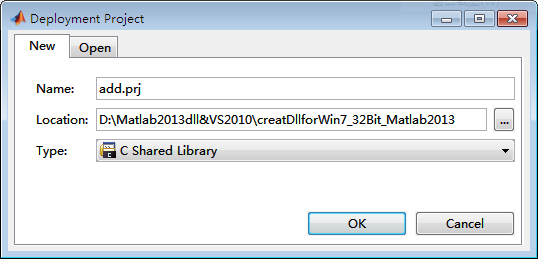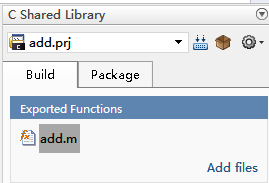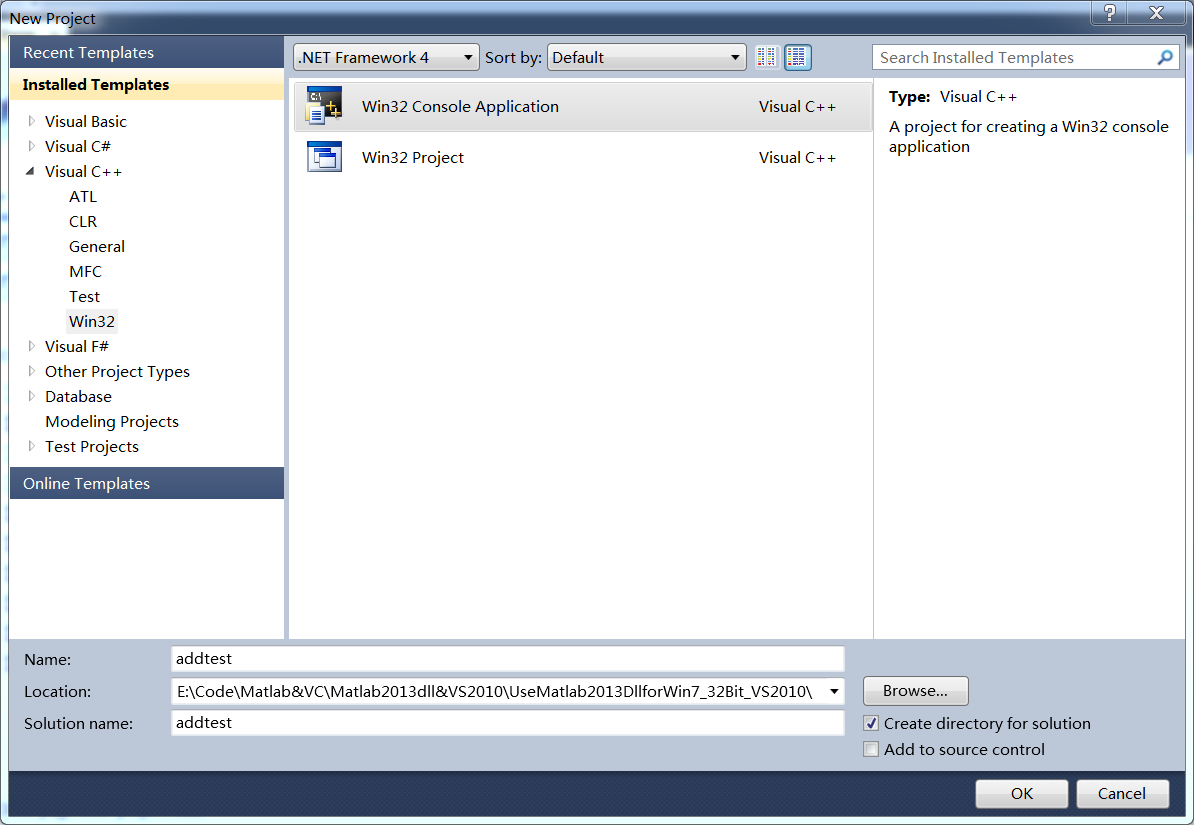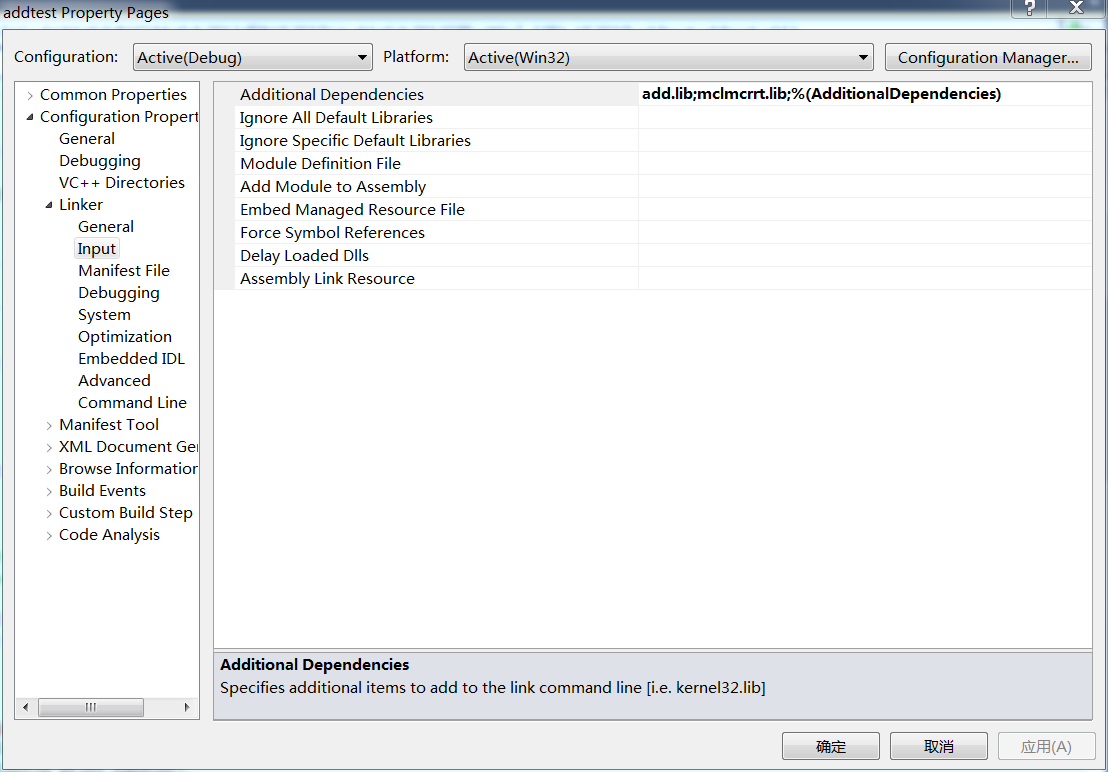第8步： 在Progect->Property->Configuration Properties->VC++ Directories（在VS2005中为Tools->Options->Projects and Solutions-> VC++ Directories）->Include Directories与Library Directories下添加路径如下图所示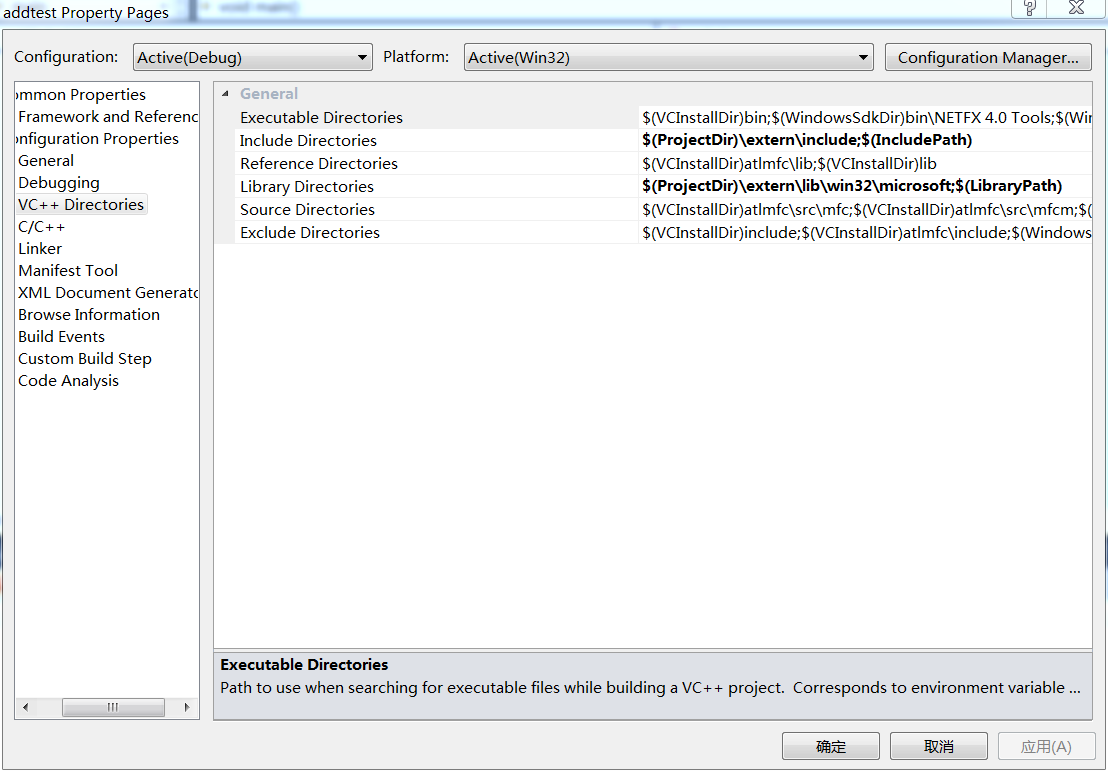#include <iostream>
#include "mclmcr.h"

using namespace std;

void main()
{
if(!mclInitializeApplication(NULL,0))  // hu 只用初始化一次
{
cout<<"初始化失败"<<endl;
system("pause");
return;
}

{
cout<<"初始化失败"<<endl;
system("pause");
return;
}

mxArray *MXa,
*MXb,
*MXcount;
mxArray *MXdata = NULL;

double a = 1,
b = 1,
count = 1E+9;
double  *data;

MXa = mxCreateDoubleMatrix(1,1,mxREAL);
memcpy(mxGetPr(MXa),&a,1*sizeof(double));
MXb = mxCreateDoubleMatrix(1,1,mxREAL);
memcpy(mxGetPr(MXb),&b,1*sizeof(double));
MXcount = mxCreateDoubleMatrix(1,1,mxREAL);
memcpy(mxGetPr(MXcount),&count,1*sizeof(double));

data = mxGetPr(MXdata);

cout<<*data<<endl;
system("pause");

mxDestroyArray(MXdata);
MXdata = 0;
mxDestroyArray(MXa);
MXa = 0;
mxDestroyArray(MXb);
MXb = 0;
mxDestroyArray(MXcount);
MXcount = 0;

mclTerminateApplication();      // hu 只用注销一次
}
展开全文• VC 调用MATLAB引擎实现可视化综合三位置铰链四杆机构的方法.pdf
• VC调用MATLAB引擎实现核反应堆复杂控制仿真的方法及应用.pdf
• 里面有m文件 说明文档 vc6的工程 ，可以直接运行的小例子，快速入门的好帮手
• VC调用MATLAB引擎实现核反应堆复杂控制仿真的方法及应用.rar
• 这是一个非常经典的实例，基于VC++编译环境下去调用matlab程序，从而实现快速，美观的作图工作，希望能帮助那些需要的人。
•   在VC中使用CPen绘制宽度大于1的虚线   Matlab的函数及指令(转) VC调用Matlab(转)  2012-01-03 14:59:06| 分类： Matlab | 标签： |字号大中小 订阅
http://wangqingyun84.blog.163.com/blog/static/7908361720120325810491/
http://wenku.baidu.com/view/77cdeaa2b0717fd5360cdc32.html
http://www.cnblogs.com/nktblog/archive/2012/04/07/2435624.html   这个博客讲的很好

VC中调用Matlab

1. Matlab与
VC混合编程的实现方法< xmlnamespace prefix ="o" ns ="urn:schemas-microsoft-com:office:office" />

Matlab作为控制系统设计的一种通用工具,它可以和VC方便的进行连接.一般而言, Matlab与VC混合编程的实现方法主要有以下三种方法:
(1) 通过引擎(Engine),采用C/S的计算模式,通过Windows的ActiveX通道和Matlab进行连接.具体应用时,往往在VC中设计程序框架,以编译的程序作为前端客户机;然后通过调用Matlab引擎在后台实现与服务器的连接,实现动态通信.
(2) 使用Matlab数学函数库. Matlab中包含了内容丰富的函数库,而且还提供了与VC的数学函数接口,用户可以方便的在VC的IDE中调用.
(3) 通过DLL实现VC与Matlab的混合编程.将Matlab中的通用功能放在DLL中,可以供多个应用程序调用.
在上述三种方法中, 第一种方法对调用Matlab的工具箱很实用,所以在进行控制系统的设计和分析时,一般都是通过调用Matlab引擎来实现的.本文主要介绍采用通过引擎来实现VC与Matlab的混合编程.

2. MATLAB引擎简介
Matlab引擎是Matlab提供的一系列程序的结合,它允许用户在自己的应用程序中对Matlab进行调用,将Matlab作为一个操作引擎使用,使其在后台运行.一般Matlab引擎采用C/S的计算方式,就是一种把应用处理负载分布到客户机和服务器上的一种计算模式,客户机和服务器既可以是存在于同一台计算机,也可以通过网络来实现信息共享.
一般情况下, 客户机是运行前端软件的PC机,并且知道如何与服务器通信,服务器与此相对应,是接收请求信息,并采取相应请求的机器.由于客户机和服务器共同承担处理负载,使系统总体性能大为提高.在实际应用过程中,把VC的C/C++语言的程序作为前端客户机程序,它从Matlab引擎接收数据信息,并与引擎传递命令的数据信息.
通过Matlab引擎用户可以完成以下任务:首先,可以将Matlab作为一个功能强大的和可编程的数学函数库,调用Matlab中的大量的数学计算函数,完成复杂的计算任务;其次,可以为一个特定的任务构建一个完整的系统,前台的用户界面用高级语言编写,后台的计算任务由Matlab引擎来完成;最后,用户不但可以在本地计算机上调用Matlab引擎,而且还可以通过网络调用其它计算机上的Matlab引擎,这样可以很好的实现资源的共享和作业的分工合作.

3. Matlab引擎实现VC和Matlab混合编程的步骤
要想在VC集成环境下利用Matlab引擎实现VC和Matlab的混合编程,一般需要经过以下几个必要的步骤:
(1) Visual C++6.0 编译环境的设置:通过菜单Tools/ Options,打开Visual C++6.0设置属性页,进入 Directorie页面,在 Show Directories for下拉列表中选择Include Files,添加路径: D:\Matlab6p5\extern\include（在Matlab的安装路径下）;再选择下拉列表中的 Library Files, 添加路径: D:\Matlab6p5\extern\ lib\win32\Microsoft\msvc60(在Matlab的安装路径下，在modelsim6.5以上的版本中，没有msv60这个文件，只添加路径到D:\Matlab6p5\extern\ lib\win32\Microsoft即可). 编译环境只需设置一次.  个人理解，类似于quartus和modlesim联合仿真时，在工具里添加modelsim的路径，///
(2) 添加库文件:通过菜单 Projects | settings,进入Link页面,在Object/Library Modules框中,添加库文件名: libmx.lib libmat.lib libeng.lib.每个新的工程文件都要单独添加库文件.  ///个人理解：类似于quartus添加test文件
(3) 在使用 engine函数的程序头文件中包含如下两个头文件:
#include "engine.h"
#include <stdlib.h>
(4) 在应用程序中添加调用Matlab引擎的程序代码:
Engine *ep;
if(!(ep=engOpen(" \0"))) //打开Matlab引擎，建立与本地Matlab的连接
{
fprintf(stderr,"\n Can't start MATLAB engine\n");
exit(-1);
}
以上步骤是VC与Matlab混合编程的编译环境进行设置,在完成了以上基本步骤后就可以利用Matlab引擎库函数engEvalString来调用Matlab的内建函数了.

4. 例子  （仿真成功了）
/*   过程分析  (不是和本程序完全对应，4.5条没有在程序中体现)   在C、C＋＋中调用Matlab的主要过程如下所示：    1） 首先调用engOpen函数打开一个Matlab工程。    2） 生成mxArray。    在Matlab中，所有变量类型，如标量、向量、矩阵、字符串、细胞矩阵和结构，都以mxArrays形式来保存，  固数据操作都必须通过这些mxArrays来完成。生成mxArray有两种不同的方式。一是用mxCreate函数来创建矩阵，  之后用mxSetName函数对它进行命名。   另一种方式是选择将一个自定义的数据结构复制到mxArray中，  值得注意的是Matlab在存储矩阵时是按列序保存的，而在C、C＋＋中是按行序保存的，所以转换时注意分清下标。   3） 调用engPutArray函数将矩阵放入到Matlab工作区中。      4） 通过engEvalString函数向Matlab传递命令，如果成功，函数返回值为此0，否则返回一个非0值。      5） 将Matlab中的运行结果回传给C、C＋＋
*/
#include <engine.h> #include <stdio.h > #include <string.h> #include <stdlib.h>  void  main ()
{
Engine *ep;
if(!(ep=engOpen(" \0"))) //调用engOpen函数打开一个Matlab工程
{ fprintf(stderr,"\n Can't start MATLAB engine\n");    exit(-1);   }
static double Areal={1,2,3,4,5,6};
mxArray *T=NULL,*a=NULL,*d=NULL;  //生成mxArray
double time={0,1,2,3,4,5,6,7,8,9};
// 利用mx函数库创建矩阵, 传递参数
T=mxCreateDoubleMatrix(1,10,mxREAL);
功能应该是建立m行n列的实双精度矩阵（mxREAL），A为mxArray数据类型。
memcpy((char*)mxGetPr(T),(char*)time,10*sizeof(double));
// 通过上面创建的Matlab Engine连接句柄将参数传递给Server端 //memcpy:void *memcpy(void *dest, const void *src, size_t n);  从源src所指的内存地址的起始位置开始拷贝n个字节到目标dest所指的内存地址的起始位置中

engPutVariable(ep,"T",T);
// 调用Matlab内建的函数和指令,调用engPutArray函数将矩阵放入到Matlab工作区中
engEvalString(ep,"D=.5.*(-9.8).*T.^5;");
engEvalString(ep,"plot(T,D);"); ///通过engEvalString函数向Matlab传递命令，如果成功，函数返回值为此0，否则返回一个非0值
}扩展：


展开全文• MATLAB在LabVIEW开发虚拟仪器中的应用研究pdf-VC调用MATLAB的方法.pdf VC调用MATLAB的方法.pdf
• 讨论了在VC调用MATLAB的3种方法, 并对3种方法进行了比较;通过MATLAB Engine方式可以调用C/C++函数和工具箱中的函数, 同时还可利用MATLAB中强大的图形功能, 但该种方式的致命缺陷是不可脱离MATLAB的环境.用LCC...
• maltab基础-基于COM的VC调用Matlab的方法及实现.pdf 附件里有...

# vc调用matlabmatlab 订阅